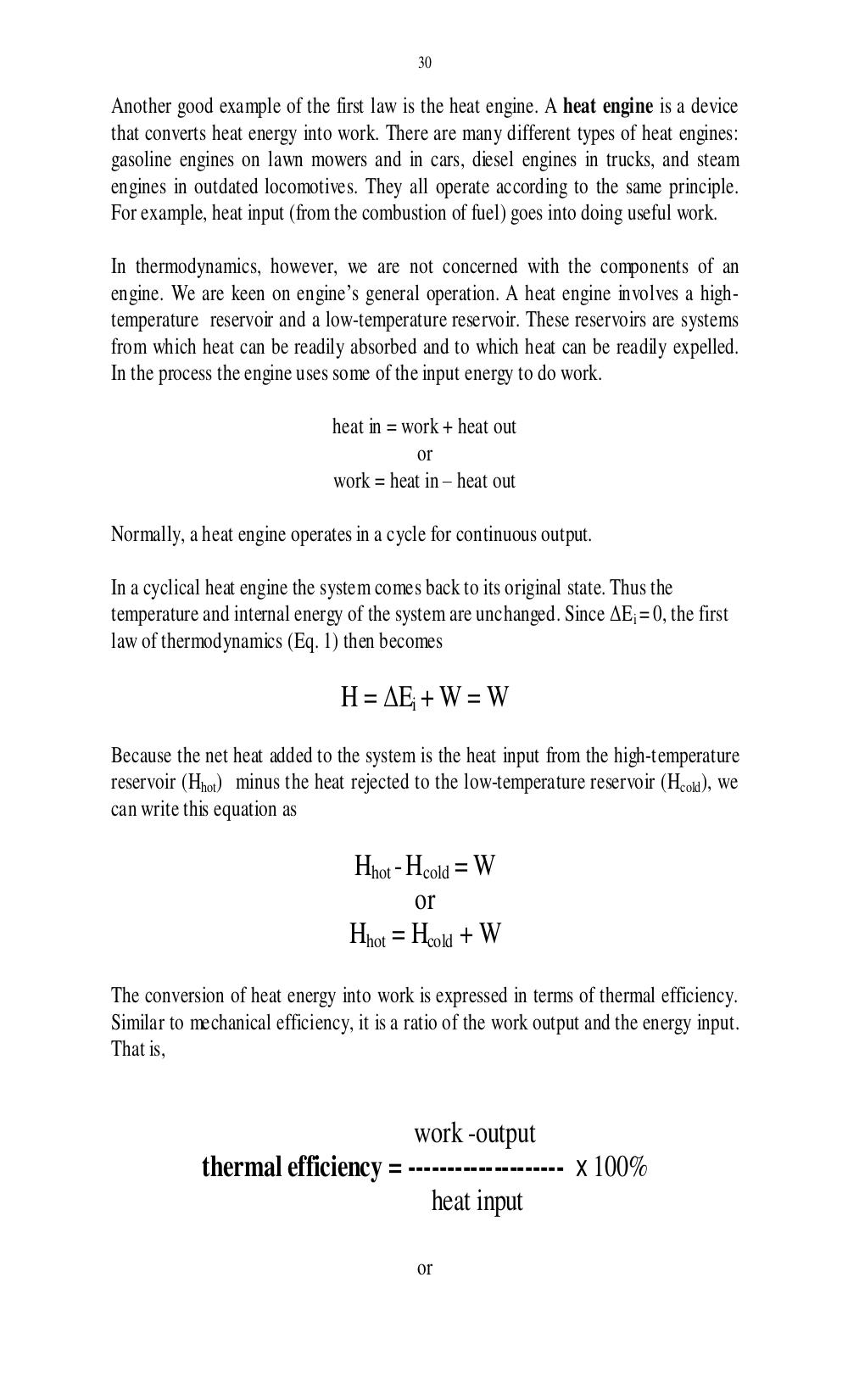# Учебно-методическое пособие по чтению специальной литературы для студентов 1 курса физического факультета. Дроздова И.В - 30 стр.

Составители:

Рубрика:

• ## Иностранный язык

30
Another good example of the first law is the heat engine. A heat engine is a device
that converts heat energy into work. There are many different types of heat engines:
gasoline engines on lawn mowers and in cars, diesel engines in trucks, and steam
engines in outdated locomotives. They all operate according to the same principle.
For example, heat input (from the combustion of fuel) goes into doing useful work.
In thermodynamics, however, we are not concerned with the components of an
engine. We are keen on engines general operation. A heat engine involves a high-
temperature reservoir and a low-temperature reservoir. These reservoirs are systems
from which heat can be readily absorbed and to which heat can be readily expelled.
In the process the engine uses some of the input energy to do work.
heat in = work + heat out
or
work = heat in heat out
Normally, a heat engine operates in a cycle for continuous output.
In a cyclical heat engine the system comes back to its original state. Thus the
temperature and internal energy of the system are unchanged. Since Δ E
i
= 0, the first
law of thermodynamics (Eq. 1) then becomes
H = Δ E
i
+ W = W
Because the net heat added to the system is the heat input from the high-temperature
reservoir (H
hot
) minus the heat rejected to the low-temperature reservoir (H
cold
), we
can write this equation as
H
hot
-
H
cold
= W
or
H
hot
= H
cold
+ W
The conversion of heat energy into work is expressed in terms of thermal efficiency.
Similar to mechanical efficiency, it is a ratio of the work output and the energy input.
That is,
work -output
thermal efficiency = -------------------- x 100%
heat input
or30

Another good example of the first law is the heat engine. A heat engine is a device
that converts heat energy into work. There are many different types of heat engines:
gasoline engines on lawn mowers and in cars, diesel engines in trucks, and steam
engines in outdated locomotives. They all operate according to the same principle.
For example, heat input (from the combustion of fuel) goes into doing useful work.

In thermodynamics, however, we are not concerned with the components of an
engine. We are keen on engine’s general operation. A heat engine involves a high-
temperature reservoir and a low-temperature reservoir. These reservoirs are systems
from which heat can be readily absorbed and to which heat can be readily expelled.
In the process the engine uses some of the input energy to do work.

heat in = work + heat out
or
work = heat in – heat out

Normally, a heat engine operates in a cycle for continuous output.

In a cyclical heat engine the system comes back to its original state. Thus the
temperature and internal energy of the system are unchanged. Since ΔE i = 0, the first
law of thermodynamics (Eq. 1) then becomes

H = ΔEi + W = W
Because the net heat added to the system is the heat input from the high-temperature
reservoir (Hhot) minus the heat rejected to the low-temperature reservoir (Hcold ), we
can write this equation as

Hhot - Hcold = W
or
Hhot = Hcold + W
The conversion of heat energy into work is expressed in terms of thermal efficiency.
Similar to mechanical efficiency, it is a ratio of the work output and the energy input.
That is,

work -output
thermal efficiency = -------------------- x 100%
heat input

or